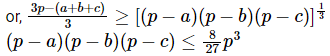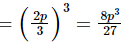a, b and c are positive real numbers such th...
a, b and c are positive real numbers such that a + b + c = p, where 'p' is a constant. Find the maximum value of (p - a) (p - b)(p - c).
• a)
(p3 - abc)
• b)
8p3/27
• c)
5p3/16
• d)
9p3/8
a, b and c are positive real numbers such that a + b + c = p, where '...
(p − a), (p − b) and (p − c) all are positive numbers.
∴ AM ≥ GMHence (b) is the correct answer.
Alternative Method:
(p − a) + (p − b) + (p − c) = 2p(constant)
⇒ (p − a)⋅(p − b)⋅(p − c) will be maximum when
p − a = p − b = p − c = 2p/3
Maximum value of the product1 Crore+ students have signed up on EduRev. Have you?
1 Crore+ students have signed up on EduRev. Have you?

### Learn this topic in detailIPMAT Mock Test - 8 (New Pattern) 100 Ques | 90 MinsMore from Related Course Quantitative Aptitude (Quant)View courses related to this question

### Quick links for CAT exam850+
Video Lectures2500+
Revision Notes600+
Online Tests10,000+
Doubts Solved
a, b and c are positive real numbers such that a + b + c = p, where 'p' is a constant. Find the maximum value of (p - a) (p - b)(p - c).a)(p3 - abc)b)8p3/27c)5p3/16d)9p3/8Correct answer is option 'B'. Can you explain this answer?
Question Description
a, b and c are positive real numbers such that a + b + c = p, where 'p' is a constant. Find the maximum value of (p - a) (p - b)(p - c).a)(p3 - abc)b)8p3/27c)5p3/16d)9p3/8Correct answer is option 'B'. Can you explain this answer? for CAT 2023 is part of CAT preparation. The Question and answers have been prepared according to the CAT exam syllabus. Information about a, b and c are positive real numbers such that a + b + c = p, where 'p' is a constant. Find the maximum value of (p - a) (p - b)(p - c).a)(p3 - abc)b)8p3/27c)5p3/16d)9p3/8Correct answer is option 'B'. Can you explain this answer? covers all topics & solutions for CAT 2023 Exam. Find important definitions, questions, meanings, examples, exercises and tests below for a, b and c are positive real numbers such that a + b + c = p, where 'p' is a constant. Find the maximum value of (p - a) (p - b)(p - c).a)(p3 - abc)b)8p3/27c)5p3/16d)9p3/8Correct answer is option 'B'. Can you explain this answer?.
Solutions for a, b and c are positive real numbers such that a + b + c = p, where 'p' is a constant. Find the maximum value of (p - a) (p - b)(p - c).a)(p3 - abc)b)8p3/27c)5p3/16d)9p3/8Correct answer is option 'B'. Can you explain this answer? in English & in Hindi are available as part of our courses for CAT. Download more important topics, notes, lectures and mock test series for CAT Exam by signing up for free.
Here you can find the meaning of a, b and c are positive real numbers such that a + b + c = p, where 'p' is a constant. Find the maximum value of (p - a) (p - b)(p - c).a)(p3 - abc)b)8p3/27c)5p3/16d)9p3/8Correct answer is option 'B'. Can you explain this answer? defined & explained in the simplest way possible. Besides giving the explanation of a, b and c are positive real numbers such that a + b + c = p, where 'p' is a constant. Find the maximum value of (p - a) (p - b)(p - c).a)(p3 - abc)b)8p3/27c)5p3/16d)9p3/8Correct answer is option 'B'. Can you explain this answer?, a detailed solution for a, b and c are positive real numbers such that a + b + c = p, where 'p' is a constant. Find the maximum value of (p - a) (p - b)(p - c).a)(p3 - abc)b)8p3/27c)5p3/16d)9p3/8Correct answer is option 'B'. Can you explain this answer? has been provided alongside types of a, b and c are positive real numbers such that a + b + c = p, where 'p' is a constant. Find the maximum value of (p - a) (p - b)(p - c).a)(p3 - abc)b)8p3/27c)5p3/16d)9p3/8Correct answer is option 'B'. Can you explain this answer? theory, EduRev gives you an ample number of questions to practice a, b and c are positive real numbers such that a + b + c = p, where 'p' is a constant. Find the maximum value of (p - a) (p - b)(p - c).a)(p3 - abc)b)8p3/27c)5p3/16d)9p3/8Correct answer is option 'B'. Can you explain this answer? tests, examples and also practice CAT tests.
Do you know? How Toppers prepare for CAT Exam
With help of the best CAT teachers & toppers, We have prepared a guide for student who are preparing for CAT : 15 Steps to clear CAT Exam(Scan QR code)